StatLect

# Cumulant generating function

The cumulant generating function of a random variable is the natural logarithm of its moment generating function.

The cumulant generating function is often used because it facilitates some calculations. In particular, its derivatives at zero, called cumulants, have interesting relations with moments and central moments.## Review of mgf

Remember that the moment generating function (mgf) of a random variableis defined asprovided that the expected value exists and is finite for allbelonging to a closed interval, with.

The mgf has the property that its derivatives at zero are equal to the moments of:The existence of the mgf guarantees that the moments (hence the derivatives at zero) exist and are finite for every.

## Definition

The cumulant generating function (cgf) is defined as follows.

Definition Suppose that a random variablepossesses a moment generating function. Then, the functionis the cumulant generating function of.

Note that the cgf is well-defined sinceis strictly positive for any.

Since the mgf completely characterizes the distribution of a random variable and the natural logarithm is a one-to-one function, also the cumulant generating function completely characterizes the distribution of a random variable.

## Cumulants

The derivatives of the cgf at zero are called cumulants.

The-th cumulant is## First three cumulants

The first cumulant is equal to the expected value:Proof

The first derivative of the cgf isSincewe haveThe second cumulant is equal to the variance:Proof

The second derivative of the cgf isWhen we evaluate it at, we getThe third cumulant is equal to the third central moment:Proof

The third derivative of the cgf is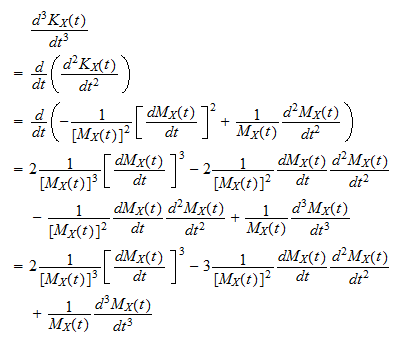When we evaluate it at, we getBut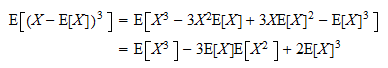Therefore,The relation of higher cumulants to moments and central moments is more complicated.

## Multivariate version

Whenis arandom vector, the joint moment generating function ofis defined as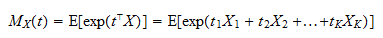provided that the expected value exists and is finite for allreal vectorsbelonging to a closed rectangle:withfor all.

The joint mgf has the property that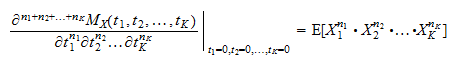The existence of the mgf guarantees the existence and finiteness of the cross-moments on the left-hand side of the equation.

Having reviewed the basic properties of the joint mgf, we are ready to define the joint cumulant generating function.

Definition Suppose that a random vectorpossesses a joint moment generating function. Then, the functionis the joint cumulant generating function of.

The definition is basically the same given for random variables.

## Cross-cumulants

The partial derivatives of the joint cgf at zero are called cross-cumulants (or joint cumulants).

Cross-cumulants are denoted as follows: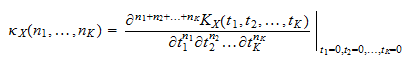## Important cross-cumulants

First-order cross-cumulants are equal to the expected values of the entries of:Proof

The first partial derivative of the joint cgf with respect toisSince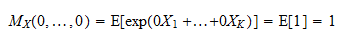we have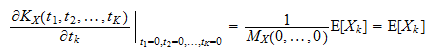Second-order joint cumulants are equal to the covariances between the entries of: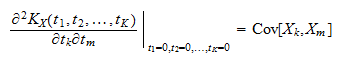Proof

The first partial derivative of the joint cgf with respect toisBy taking the second derivative with respect to, we obtain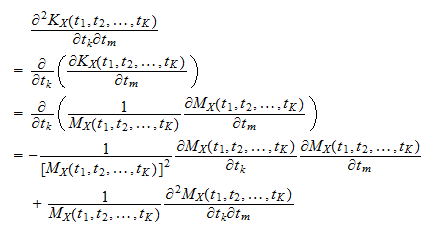Sincewe have